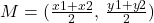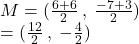## Find the midpoint of the line segment joining points A and B. A(6,- 7); B(6,3) The midpoint of the line segment is (Type a

Question

Find the midpoint of the line segment joining points A and B.
A(6,- 7); B(6,3)
The midpoint of the line segment is
(Type an ordered pair.)

in progress 0
5 months 2021-08-28T11:24:27+00:00 1 Answers 3 views 0

## ( 6 , – 2)

Step-by-step explanation:

The midpoint M of two endpoints of a line segment can be found by using the formulawhere

(x1 , y1) and (x2 , y2) are the points

From the question the points are

A(6,- 7); B(6,3)

The midpoint isWe have the final answer as

### ( 6 , – 2)

Hope this helps you